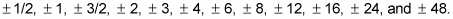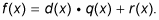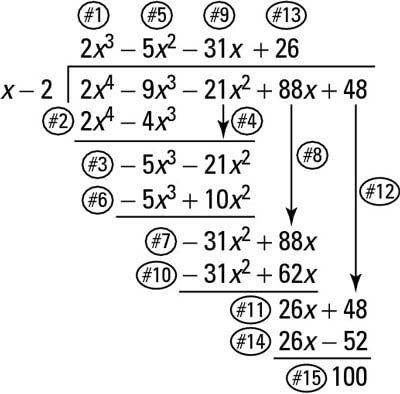##### Pre-Calculus: 1001 Practice Problems For Dummies (+ Free Online Practice)Once you have used the rational root theorem to list all the possible rational roots of any polynomial, the next step is to test the roots. One way is to use long division of polynomials and hope that when you divide you get a remainder of 0. Once you have a list of possible rational roots, you then pick one and assume that it’s a root.

For example, consider the equation f(x) = 2x4 – 9x3 – 21x2 + 88x + 48, which has the following possible rational roots:If x = c is a root, then x – c is a factor. So if you pick x = 2 as your guess for the root, x – 2 should be a factor. You can use long division to test if x – 2 is actually a factor and, therefore, x = 2 is a root.

Dividing polynomials to get a specific answer isn’t something you do every day, but the idea of a function or expression that’s written as the quotient of two polynomials is important for pre-calculus. If you divide a polynomial by another and get a remainder of 0, the divisor is a factor, which in turn gives a root.

In math lingo, the division algorithm states the following: If f(x) and d(x) are polynomials such that d(x) isn’t equal to 0, and the degree of d(x) isn’t larger than the degree of f(x), there are unique polynomials q(x) and r(x) such thatIn plain English, the dividend equals the divisor times the quotient plus the remainder. You can always check your results by remembering this information.

Remember the mnemonic device Dirty Monkeys Smell Bad when doing long division to check your roots. Make sure all terms in the polynomial are listed in descending order and that every degree is represented. In other words, if x2 is missing, put in a placeholder of 0x2 and then do the division. (This step is just to make the division process easier.)

To divide two polynomials, follow these steps:

1. Divide.

Divide the leading term of the dividend by the leading term of the divisor. Write this quotient directly above the term you just divided into.

2. Multiply.

Multiply the quotient term from Step 1 by the entire divisor. Write this polynomial under the dividend so that like terms are lined up.

3. Subtract.

Subtract the whole line you just wrote from the dividend.

You can change all the signs and add if it makes you feel more comfortable. This way, you won’t forget signs.

4. Bring down the next term.

Do exactly what this says; bring down the next term in the dividend.

5. Repeat Steps 1–4 over and over until the remainder polynomial has a degree that’s less than the dividend’s.

The following list explains how to divide 2x4 – 9x3 – 21x2 + 88x + 48 by x – 2. Each step corresponds with the numbered step in the illustration in this figure.The process of long division of polynomials.

(Note that using Descartes’s rule of signs, you find that this particular example may have positive roots, so it’s efficient to try a positive number here. If Descartes’s rule of signs had said that no positive roots existed, you wouldn’t test any positives!)

1. Divide.

What do you have to multiply x in the divisor by to make it become 2x4 in the dividend? The quotient, 2x3, goes above the 2x4 term.

2. Multiply.

Multiply this quotient by the divisor and write it under the dividend.

3. Subtract.

Subtract this line from the dividend: (2x4 – 9x3) – (2x4 – 4x3) = –5x3. If you’ve done the job right, the subtraction of the first terms always produces 0.

4. Bring down.

Bring down the other terms of the dividend.

5. Divide.

What do you have to multiply x by to make it –5x3? Put the answer, –5x2, above the –21x2.

6. Multiply.

Multiply the –5x2 times the x – 2 to get –5x3 + 10x2. Write it under the remainder with the degrees lined up.

7. Subtract.

You now have (–5x3 – 21x2) – (–5x3 + 10x2) = –31x2.

8. Bring down.

The +88x takes its place.

9. Divide.

What to multiply by to make x become –31x2? The quotient –31x goes above –21x2.

10. Multiply.

The value –31x times (x – 2) is –31x2 + 62x; write it under the remainder.

11. Subtract.

You now have (–31x2 + 88x) – (–31x2 + 62x), which is 26x.

12. Bring down.

The +48 comes down.

13. Divide.

The term 26x divided by x is 26. This answer goes on top.

14. Multiply.

The constant 26 multiplied by (x – 2) is 26x – 52.

15. Subtract.

You subtract (26x + 48) – (26x – 52) to get 100.

16. Stop.

The remainder 100 has a degree that’s less than the divisor of x – 2.

Wow . . . now you know why they call it long division. You went through all that to find out that x – 2 isn’t a factor of the polynomial, which means that x = 2 isn’t a root.

If you divide by c and the remainder is 0, then the linear expression (xc) is a factor and that c is a root. A remainder other than 0 implies that (xc) isn’t a factor and that c isn’t a root.